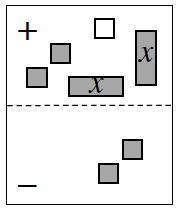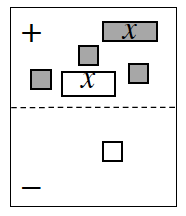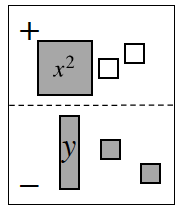### Home > INT1 > Chapter A > Lesson A.1.3 > ProblemA-30

A-30.

Write a simplified algebraic expression for each Expression Mat below.1.Remove the zero pairs.
What is an expression for the remaining tiles?

$2x − 1$

1.On this mat, both $x$-tiles in the (+) region can be removed, since they make a zero pair.
Next, flip the $−1$ unit tile in the (-) region to the (+) region, and then simplify.

1.Unlike in parts (a) and (b), this problem has no zero pairs.
However, here is an un-simplified expression to get you started.
$x^{2} + \left(−1\right) + \left(−1\right) − \left(y + 1 + 1\right)$
Can you simplify that expression?